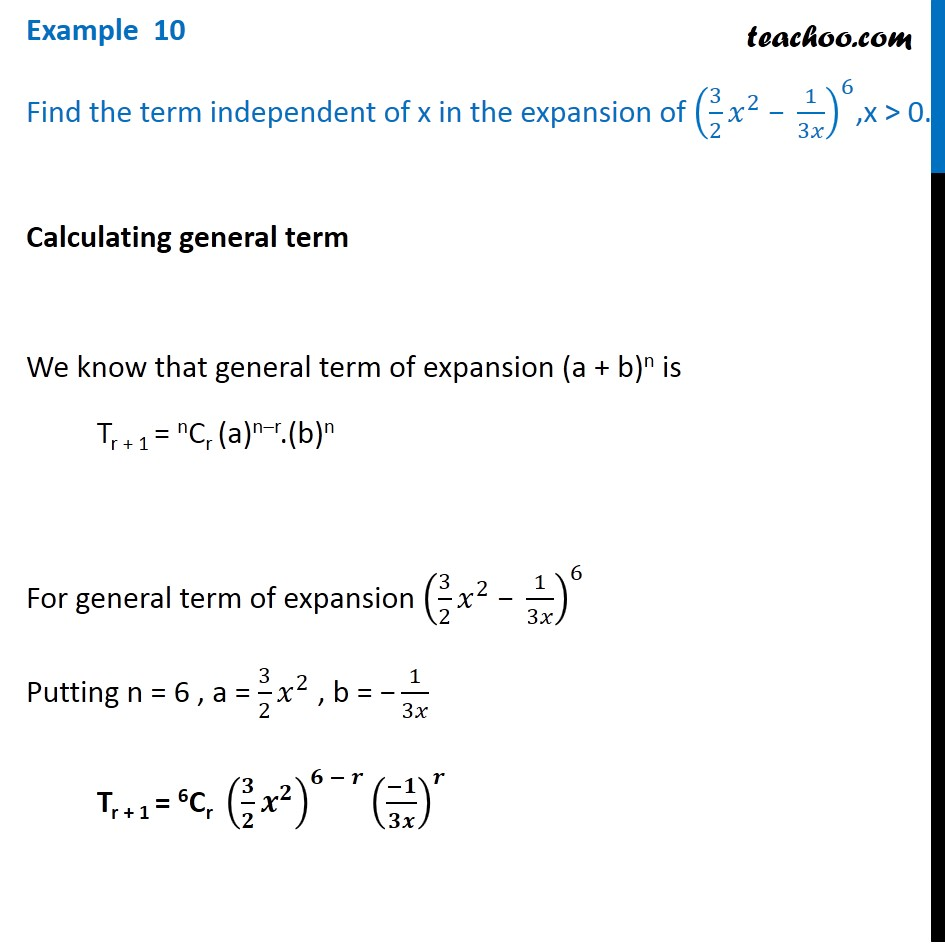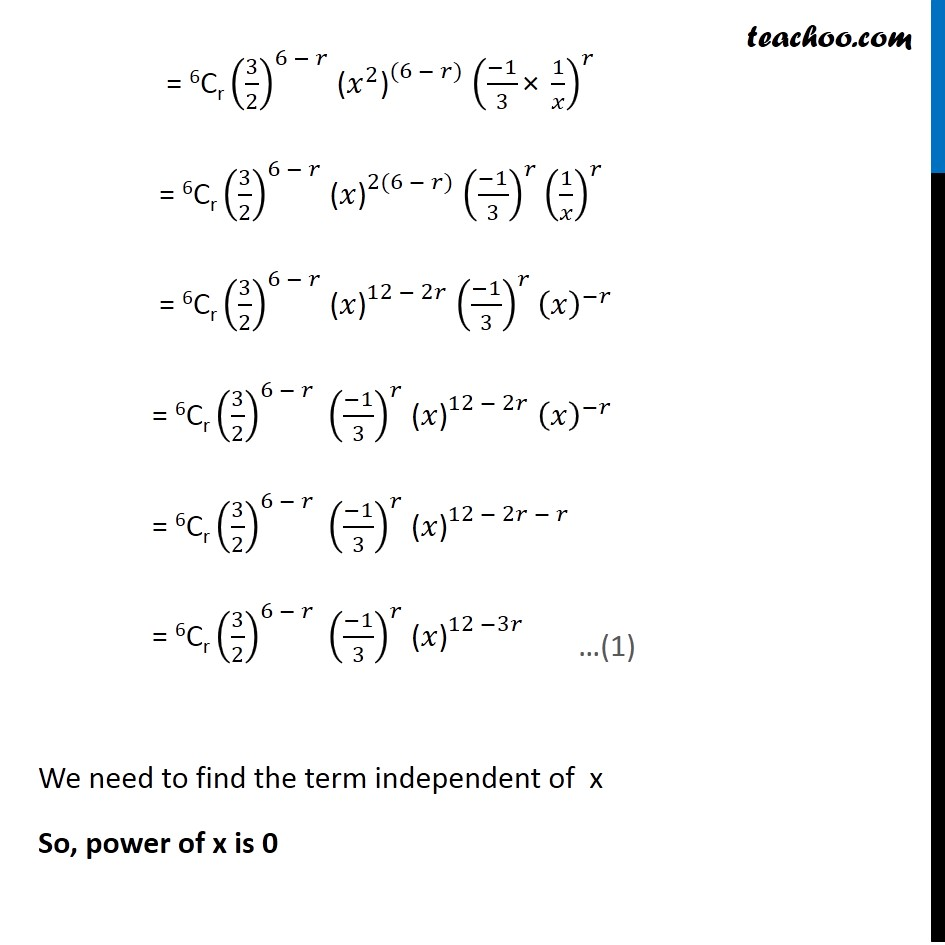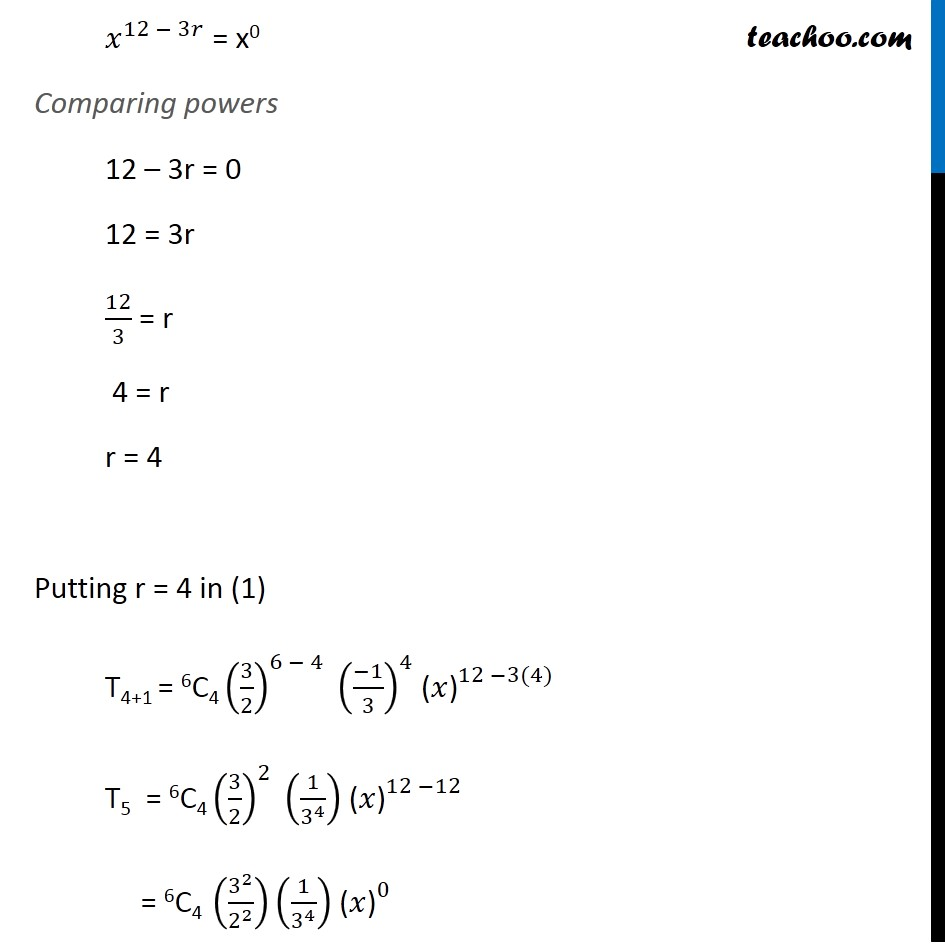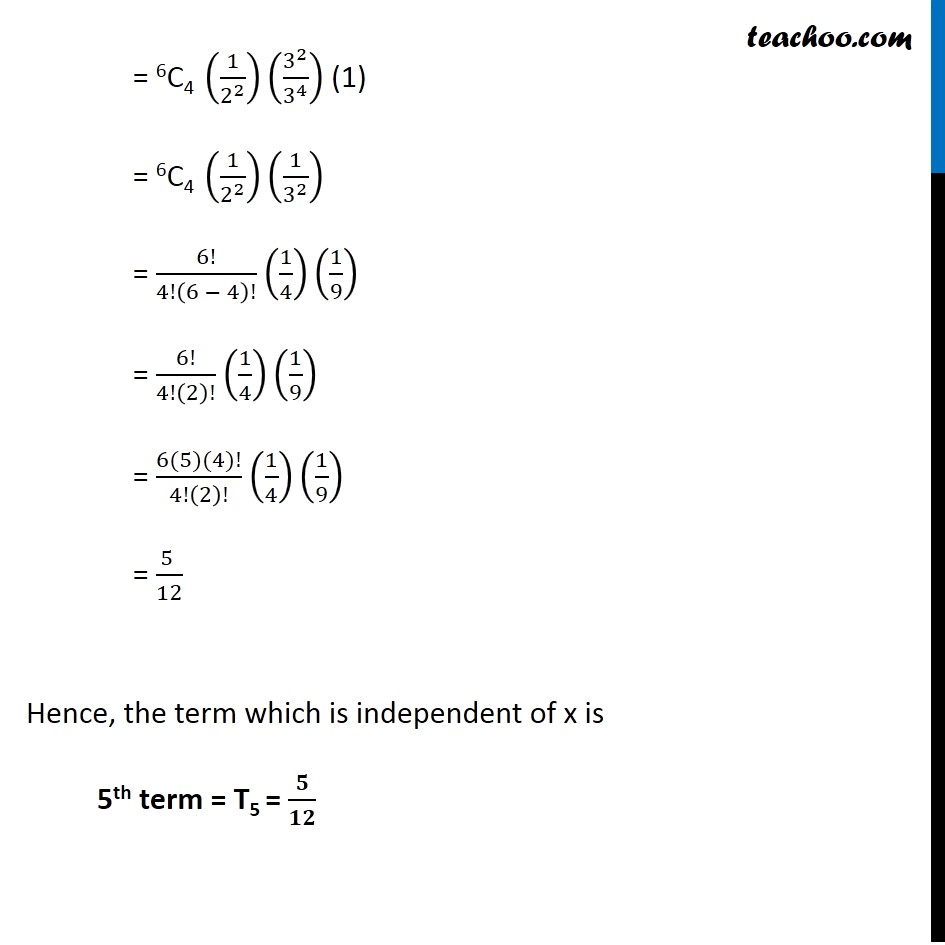Examples

Chapter 7 Class 11 Binomial Theorem
Serial order wiseLearn in your speed, with individual attention - Teachoo Maths 1-on-1 Class

### Transcript

Question 6 Find the term independent of x in the expansion of (3/2 𝑥^2 " − " 1/3𝑥)^6,x > 0. Calculating general term We know that general term of expansion (a + b)n is Tr + 1 = nCr (a)n–r.(b)n For general term of expansion (3/2 𝑥^2 " − " 1/3𝑥)^6 Putting n = 6 , a = 3/2 𝑥^2 , b = "−" 1/3𝑥 Tr + 1 = 6Cr (𝟑/𝟐 𝒙^𝟐 )^(𝟔 − 𝒓) ((−𝟏)/𝟑𝒙)^𝒓 = 6Cr (3/2)^(6 − 𝑟) (𝑥^2 ")" ^((6 − 𝑟)) ((−1)/3 "×" 1/𝑥)^𝑟 = 6Cr (3/2)^(6 − 𝑟) (𝑥")" ^(2(6 − 𝑟)) ((−1)/3)^𝑟 (1/𝑥)^𝑟 = 6Cr (3/2)^(6 − 𝑟) (𝑥")" ^(12 − 2𝑟) ((−1)/3)^𝑟 (𝑥)^(−𝑟) = 6Cr (3/2)^(6 − 𝑟) ((−1)/3)^𝑟 (𝑥")" ^(12 − 2𝑟) (𝑥)^(−𝑟) = 6Cr (3/2)^(6 − 𝑟) ((−1)/3)^𝑟 (𝑥")" ^(12 − 2𝑟 − 𝑟) = 6Cr (3/2)^(6 − 𝑟) ((−1)/3)^𝑟 (𝑥")" ^(12 −3𝑟) We need to find the term independent of x So, power of x is 0 𝑥^(12 − 3𝑟) = x0 Comparing powers 12 – 3r = 0 12 = 3r 12/3 = r 4 = r r = 4 Putting r = 4 in (1) T4+1 = 6C4 (3/2)^(6 − 4) ((−1)/3)^4 (𝑥")" ^(12 −3(4)) T5 = 6C4 (3/2)^2 (1/3^4 ) (𝑥")" ^(12 −12) = 6C4 (3^2/2^2 )(1/3^4 ) (𝑥")" ^0 = 6C4 (1/2^2 )(3^2/3^4 ) (1) = 6C4 (1/2^2 )(1/3^2 ) = 6!/4!(6 − 4)! (1/4)(1/9) = 6!/4!(2)! (1/4)(1/9) = (6(5)(4)!)/4!(2)! (1/4)(1/9) = (5 )/12 Hence, the term which is independent of x is 5th term = T5 = 𝟓/𝟏𝟐